# DC Mesh Current Analysis

## Network Analysis Techniques

• #### Question 1

 Don’t just sit there! Build something!!

Learning to mathematically analyze circuits requires much study and practice. Typically, students practice by working through lots of sample problems and checking their answers against those provided by the textbook or the instructor. While this is good, there is a much better way.

You will learn much more by actually building and analyzing real circuits, letting your test equipment provide the answers” instead of a book or another person. For successful circuit-building exercises, follow these steps:

1. Carefully measure and record all component values prior to circuit construction.
2. Draw the schematic diagram for the circuit to be analyzed.
3. Carefully build this circuit on a breadboard or other convenient medium.
4. Check the accuracy of the circuit’s construction, following each wire to each connection point, and verifying these elements one-by-one on the diagram.
5. Mathematically analyze the circuit, solving for all values of voltage, current, etc.
6. Carefully measure those quantities, to verify the accuracy of your analysis.
7. If there are any substantial errors (greater than a few percent), carefully check your circuit’s construction against the diagram, then carefully re-calculate the values and re-measure.

Avoid very high and very low resistor values, to avoid measurement errors caused by meter “loading”. I recommend resistors between 1 kΩ and 100 kΩ, unless, of course, the purpose of the circuit is to illustrate the effects of meter loading! One way you can save time and reduce the possibility of error is to begin with a very simple circuit and incrementally add components to increase its complexity after each analysis, rather than building a whole new circuit for each practice problem. Another time-saving technique is to re-use the same components in a variety of different circuit configurations. This way, you won’t have to measure any component’s value more than once.

• #### Question 2

A transistor is a semiconductor device that acts as a constant-current regulator. For the sake of analysis, transistors are often considered as constant-current sources: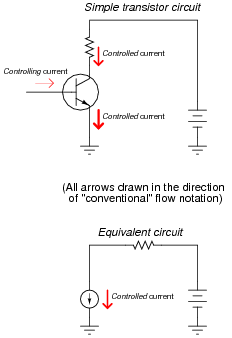Suppose we needed to calculate the amount of current drawn from the 6-volt source in this dual-source transistor circuit:We know the combined currents from the two voltage sources must add up to 5 mA, because Kirchhoff’s Current Law tells us that currents add algebraically at any node. Based on this knowledge, we may label the current through the 6-volt battery as “I”, and the current through the 7.2 volt battery as “5 mA − I”: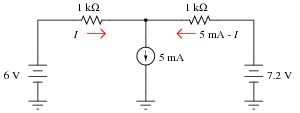Kirchhoff’s Voltage Law tells us that the algebraic sum of voltage drops around any “loop” in a circuit must equal zero. Based on all this data, calculate the value of I: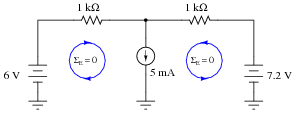Hint: simultaneous equations are not needed to solve this problem!

• #### Question 3

This transistor circuit is powered by two different voltage sources, one that outputs 6 volts, and the other that is variable.Transistors naturally act as current-regulating devices, and are often analyzed as though they were current sources. Suppose that this transistor happened to be regulating current at a value of 3.5 mA:How high does the voltage of the variable source have to be adjusted, until no current is drawn from the 6-volt battery?

Hint: simultaneous equations are not needed to solve this problem!

• #### Question 4

Write two KVL loop equations for this circuit, using I1 and I2 as the only variables:• #### Question 5

Describe, step-by-step, the steps required to calculate all currents and voltage drops in a DC network using the Mesh Current Method.

• #### Question 6

Write the KVL equations for this circuit, given the following mesh current directions, and then solve for the charging current through battery #1: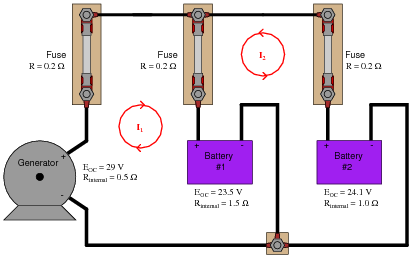Now, write the KVL equations for the same circuit, after reversing the direction of mesh current I2. How does this reversal of mesh current I2 affect the writing of the two KVL equations, and also the calculation of the answer for battery #1’s charging current?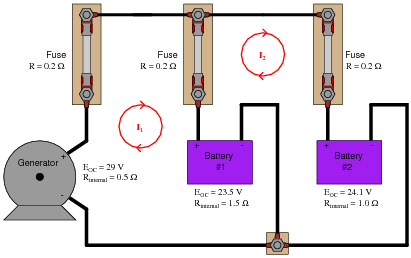• #### Question 7

The “Mesh Current” method of network analysis works well to calculate currents in unbalanced bridge circuits. Take this circuit, for example:Write three mesh equations for this circuit, following these three mesh currents: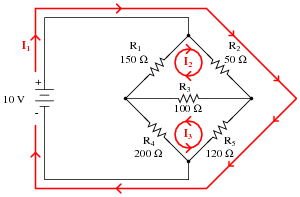• #### Question 8

A very interesting style of voltage divider appeared in an issue of Electronics, May 10, 1973. It used three series-connected strings of resistors and connection clips to provide 1000 steps of voltage division with only 31 resistors, of only 3 different resistance values: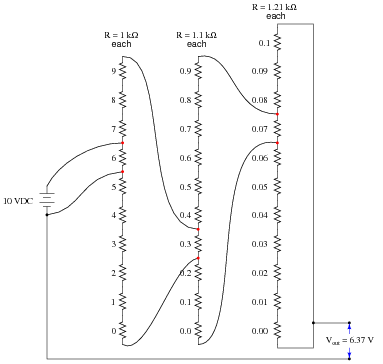By moving the connection points between these strings of resistors, different fractions of the input voltage may be obtained at the output:For the purposes of analysis, we may simplify any given configuration of this voltage divider circuit into a network of fewer resistors, in this form: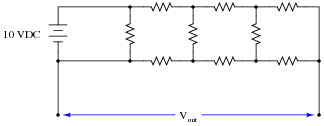Draw the simplified networks for each of the two given configurations (Vout = 6.37 volts and Vout = 2.84 volts), showing all resistance values, and then apply mesh current analysis to verify the given output voltages in each case.

Note: you will have to solve a set of simultaneous equations: 4 equations with 4 unknowns, in order to obtain each answer. I strongly recommend you use a scientific calculator to perform the necessary arithmetic!